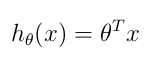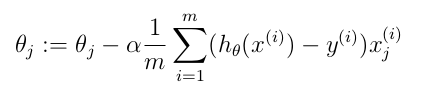# Regressão linear

Categoria: Machine Learning
Publicado em 11 de Julho de 2014

### Cost FunctionWhere:

• m: number of training sets
• h theta: hipothesis function (see instructions below)
• x: matrix where each columns represents a feature. Each line of the matrix is a training set. Number of lines of this matrix is equal to m.
• y: vector with expected value for each training set. Number of lines of this vector is equal to m.

Notes:

• x to the power of (i) means the ith line of matrix m
• y to the power of (i) means the ith element of vector y

### Hipothesis functionWhere:

• theta is a vector with number of elements equal to number of features
• x: matrix where each columns represents a feature. Each line of the matrix is a training set. Number of lines of this matrix is equal to m

Notes:

• theta to the power of T is the transpose of vector theta
• the multiplication above is a matrix multiplication
• the result of this function is a real numberWhere:

• j: value from 1..[number of features]
• theta: is a vector with number of elements equal to number of features
• alpha: learning rate. It is not possible to predict the best value for alpha. You should run the calculus a few times to discover the best value.
• m: number of training sets
• h theta: hipothesis function (see instructions above)
• x: matrix where each columns represents a feature. Each line of the matrix is a training set. Number of lines of this matrix is equal to m.
• y: vector with expected value for each training set. Number of lines of this vector is equal to m.

Notes:

• x to the power of (i) means the ith line of matrix m
• y to the power of (i) means the ith element of vector y